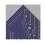# Summation Series to Product Series Part 2.

In the first part of these notes we showed the following $S(n) = \sum_{k = 1}^{k = n}\frac{f(k)}{k!} = \prod_{k = 2}^{k = n} (1 + \frac{f(k)}{a_k}) = P(n)$ for all $n > 1$ where the sequence $(a_k)$ is given by the recurrence relation $a_2 = 2$ and $a_{k+1} = (k+1)(a_k + f(k))$.

In this note we'll have a look at some applications of this.

Part 1 : A couple of Examples

For this constant function we have $S(n) = \sum_{k = 1}^{k = n}\frac{1}{k!}$. So $\prod_{k = 2}^{k \rightarrow \infty} (1 + \frac{1}{a_k}) = e-1$ where $a_2 = 2$ and $a_{k+1} = (k+1)(a_k +1)$ by considering Taylor expansion of $e$.

Here is another example : we can write the harmonic series as $\sum_{n=1}^{n \rightarrow \infty} \frac{1}{n}$. So $\prod_{k = 2}^{k \rightarrow \infty} (1 + \frac{(k-1)!}{a_k})$ where $a_2 = 2$ and $a_{k+1} = (k+1)(a_k +(k-1)!)$

Part 2 : Questions and Remarks for Brilliant Here's some questions I have for the Brilliant Community.

1) We showed that we can re-write the harmonic series as $\prod_{k = 2}^{k \rightarrow \infty} (1 + \frac{(k-1)!}{a_k})$. Can we prove that this diverges without assuming the summation series $\sum_{n=1}^{n \rightarrow \infty} \frac{1}{n}$?

2) Can we re-write the sequence $a_2=2$ and $a_{k+1} = (k+1)(a_k +f(k))$ with out recursion? Do these recursive sequences have any combinotorical properties?

3) We haven't really learnt anything significantly new from re-writing these series and in product series form this is computationally difficult due to the recursive sequences.

4) Post any interesting examples you can re-write below!Note by Roberto Nicolaides
6 years, 5 months ago

This discussion board is a place to discuss our Daily Challenges and the math and science related to those challenges. Explanations are more than just a solution — they should explain the steps and thinking strategies that you used to obtain the solution. Comments should further the discussion of math and science.

When posting on Brilliant:

• Use the emojis to react to an explanation, whether you're congratulating a job well done , or just really confused .
• Ask specific questions about the challenge or the steps in somebody's explanation. Well-posed questions can add a lot to the discussion, but posting "I don't understand!" doesn't help anyone.
• Try to contribute something new to the discussion, whether it is an extension, generalization or other idea related to the challenge.

MarkdownAppears as
*italics* or _italics_ italics
**bold** or __bold__ bold
- bulleted- list
• bulleted
• list
1. numbered2. list
1. numbered
2. list
Note: you must add a full line of space before and after lists for them to show up correctly
paragraph 1paragraph 2

paragraph 1

paragraph 2

[example link](https://brilliant.org)example link
> This is a quote
This is a quote
    # I indented these lines
# 4 spaces, and now they show
# up as a code block.

print "hello world"
# I indented these lines
# 4 spaces, and now they show
# up as a code block.

print "hello world"
MathAppears as
Remember to wrap math in $$ ... $$ or $ ... $ to ensure proper formatting.
2 \times 3 $2 \times 3$
2^{34} $2^{34}$
a_{i-1} $a_{i-1}$
\frac{2}{3} $\frac{2}{3}$
\sqrt{2} $\sqrt{2}$
\sum_{i=1}^3 $\sum_{i=1}^3$
\sin \theta $\sin \theta$
\boxed{123} $\boxed{123}$